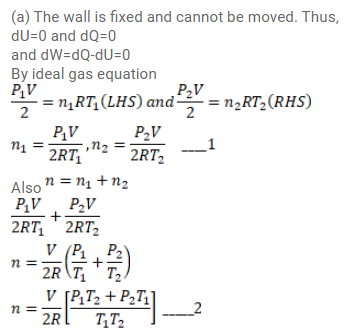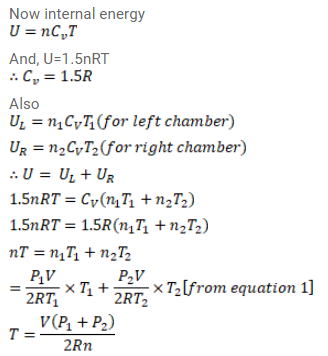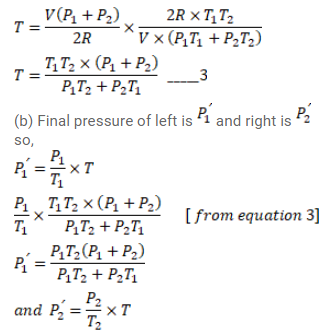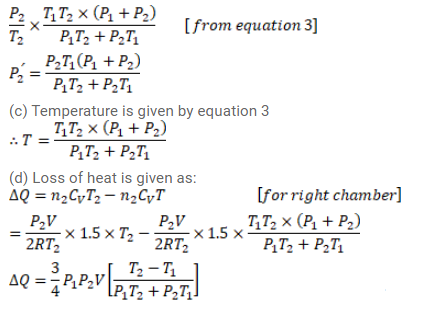# Figure (26-E11) shows a cylindrical tube of volume V

Question:

Figure (26-E11) shows a cylindrical tube of volume $\mathrm{V}$ with adiabatic walls containing an ideal gas. The internal energy of this ideal gas is given by $1.5 \mathrm{nRT}$. The tube is divided into two equal parts by a fixed diathermic wall. Initially, the pressure and the temperature are $\mathrm{p}_{1}, \mathrm{~T}_{1}$ on the left and $\mathrm{p}_{2}, \mathrm{~T}_{2}$ on the right. The system is left for sufficient time so that the temperature becomes equal on the two sides. (a) How much work has been done by the gas on the left part? (b) Find the final pressures on the two sides. (c) Find the final equilibrium temperature. (d) How much heat has flown from the gas on the right to the gas on the left?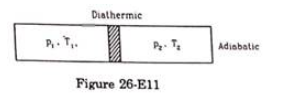Solution: